# Creating a content-based movie recommender system in Jupyter NotebookThis tutorial shows how to build a content-based movie recommender system. The output function will be able to suggest the n most similar movies to the one given as an input, based on a dataset that will be used to build the algorithm itself.

The following tutorial assumes you have Spell account and are logged in. Get \$10 GPU credit when you sign up for a new account.

## Setup Jupyter Workspace

1. Login into the Spell Web Panel https://web.spell.ml and click on Workspaces > Create Workspace.

2. Give a name to your Workspace like movie_recommender. We don’t need to add data or code from other sources, so we can leave the Add Code section empty.3. Now we select the Machine Type and Framework for which the default values are fine, the type of Jupyter setup we want to work in, and finally the packages we need to pip install: insert rake_nltk.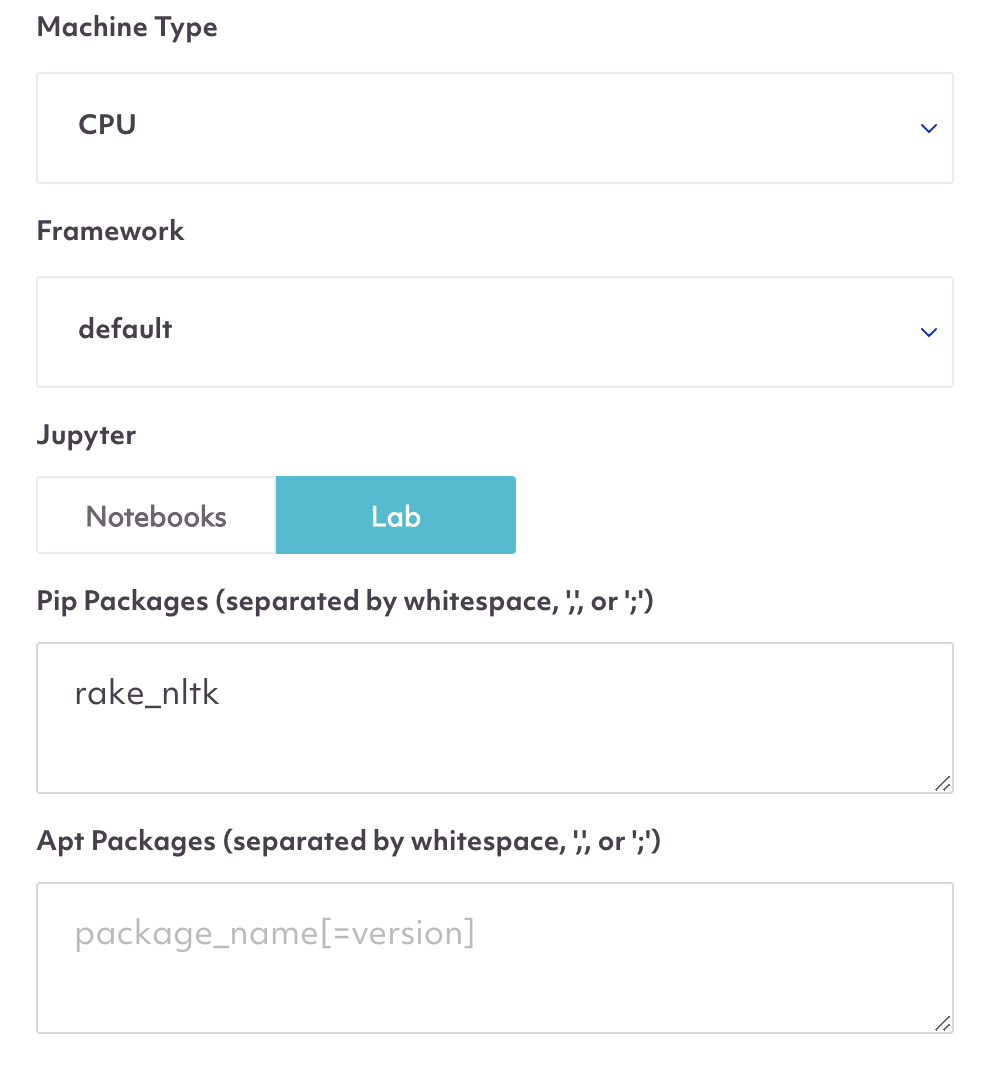`rake_nltk`

## Create movie-recommender in the Jupyter Notebook

### 1. Create Jupyter Notebook and import libraries and gather the data

We first create a new Jupyter Notebook, import the libraries we need, and set up pandas so we can see up to 100 columns of any dataframe stored in memory.

Then we read the data from a URL, which in this case is a dataset including the top 250 rated movies according to IMDB website.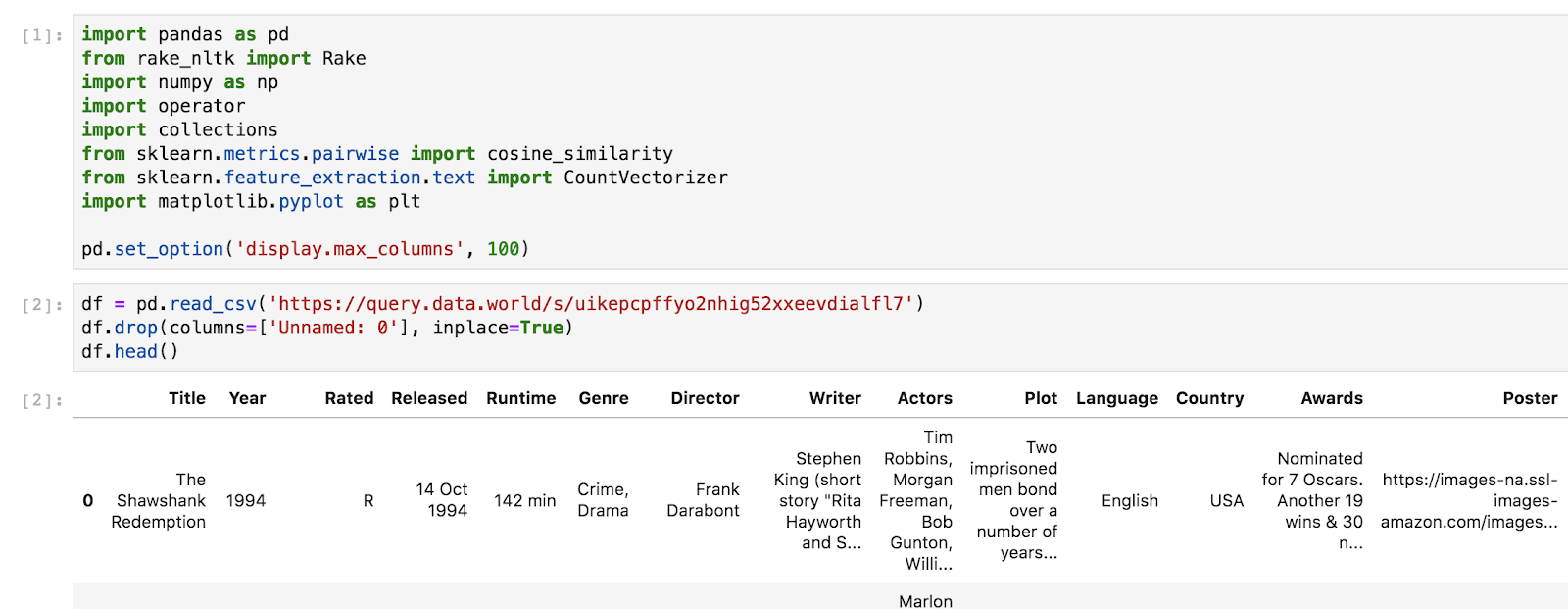```import pandas as pd
from rake_nltk import Rake
import numpy as np
import operator
import collections
from sklearn.metrics.pairwise import cosine_similarity
from sklearn.feature_extraction.text import CountVectorizer
import matplotlib.pyplot as plt```
```pd.set_option('display.max_columns', 100)
df.drop(columns=['Unnamed: 0'], inplace=True)

### 2. Cleaning the data

The dataset includes lots of information about the movies, but the similarity values will be calculated based on Genre, Director, Actors and Plot. These are the only columns we will keep, along with the Title, naturally. Note that the features’ choice is not uniquely determined, you are free to include more or fewer columns, according to what you think similarities are based on.

We select the above mentioned columns and change their names to lower-case, because it’s best practice.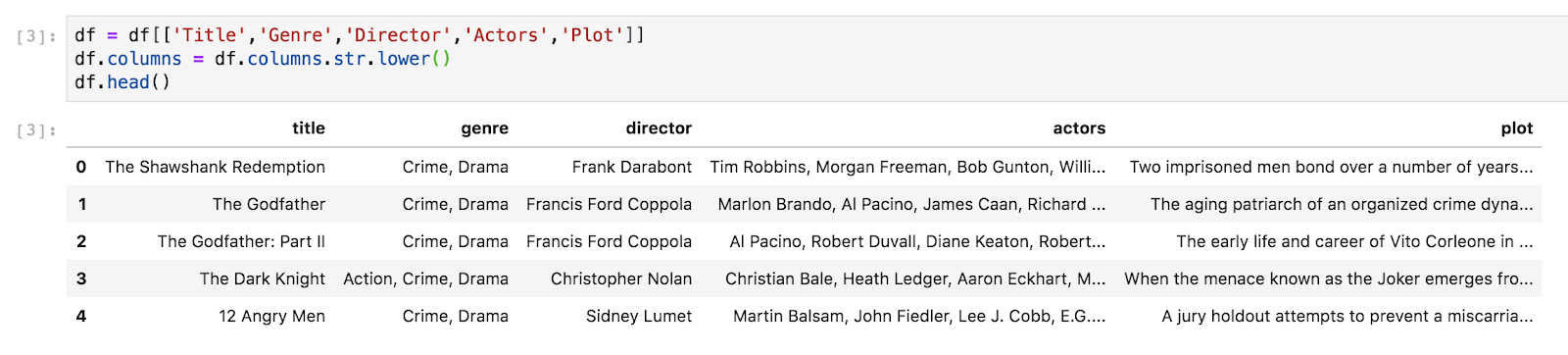```df = df[['Title','Genre','Director','Actors','Plot']]
df.columns = df.columns.str.lower()

Now it’s time to clean/manipulate the data. Since the first and last name of a person are a unique characteristic, we merge them, in the director and actors’ columns. Also, we keep the first 3 actors only because we want just the actors who play the main characters to be included in the similarity calculation. In this case as well, if you want to keep fewer or more names, you should do so.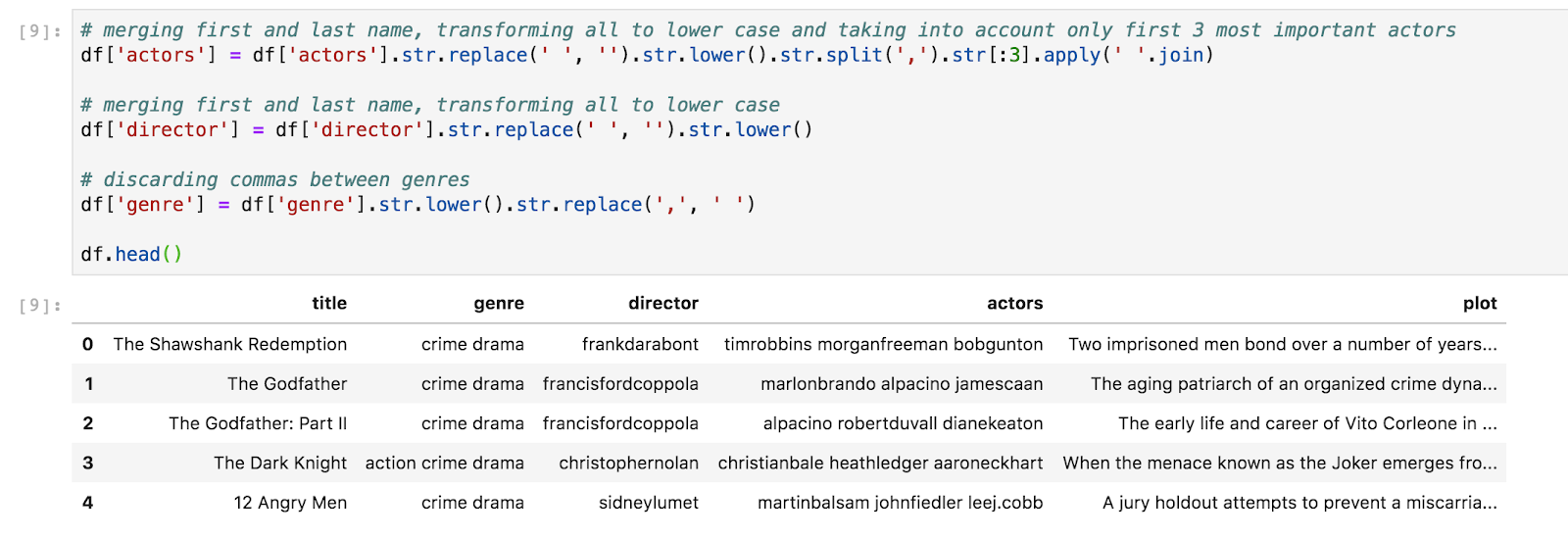```df['actors'] = df['actors'].str.replace(' ', '').str.lower().str.split(',').str[:3].apply(' '.join)

df['director'] = df['director'].str.replace(' ', '').str.lower()

df['genre'] = df['genre'].str.lower().str.replace(',', ' ')

```

We still need to clean the plot column, since we don’t want to keep the entire description of the movie, but instead we want to extract only the relevant words that describe the plot. In order to do so, we use the Rake class imported at the top of the notebook, and we write a function that will be applied to the plot column. The Rake class allows to extract key-words from a string, assigning them a score based on the importance every single word has in that context. The function below, given a string as input, will output the dictionary of the key-words (words as keys and scores as values) and the top half key-words, which is what we want to keep as feature in our similarity calculation. Finally, we set the title column as index.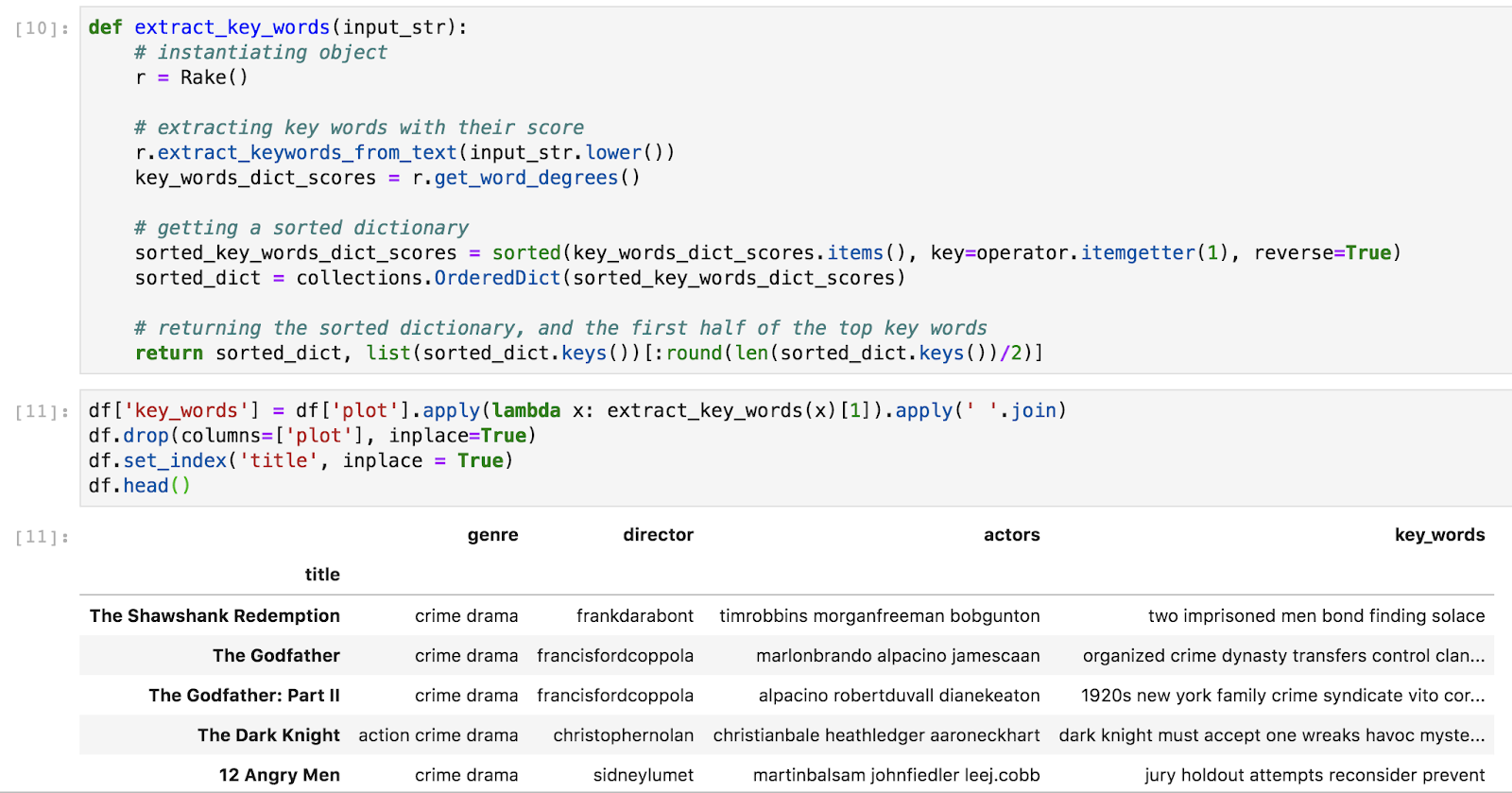```def extract_key_words(input_str):
r = Rake()
r.extract_keywords_from_text(input_str.lower())
key_words_dict_scores = r.get_word_degrees()
sorted_key_words_dict_scores = sorted(key_words_dict_scores.items(), key=operator.itemgetter(1), reverse=True)
sorted_dict = collections.OrderedDict(sorted_key_words_dict_scores)
return sorted_dict, list(sorted_dict.keys())[:round(len(sorted_dict.keys())/2)]```
```df['key_words'] = df['plot'].apply(lambda x: extract_key_words(x)).apply(' '.join)
df.drop(columns=['plot'], inplace=True)
df.set_index('title', inplace = True)

At this point, we put all the movie info in a unique column. We call this newly created dataframe, bag_of_words.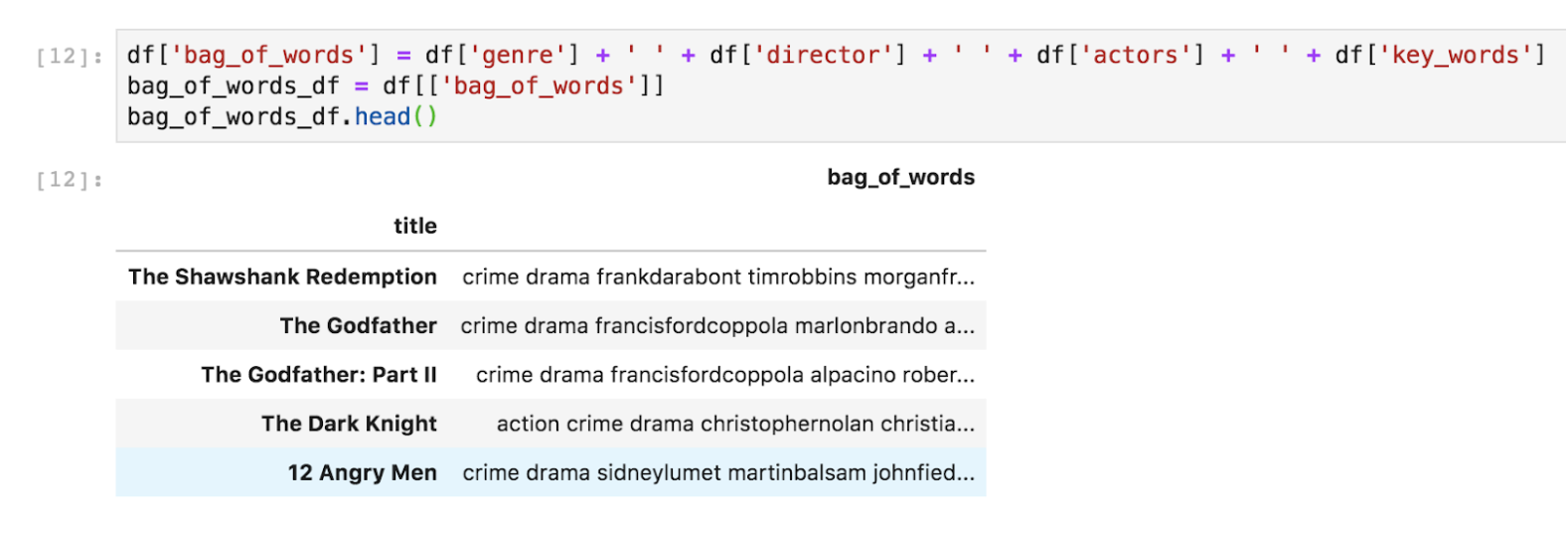```df['bag_of_words'] = df['genre'] + ' ' + df['director'] + ' ' + df['actors'] + ' ' + df['key_words']
bag_of_words_df = df[['bag_of_words']]

## 3. Modeling

Now that our bag of words is ready, we need to vectorize each row so we can calculate similarity values between couples of movies. In order to do this, we can apply CountVectorizer to the bag_of_words_df dataframe. This will create a matrix that takes all the words in the bag_of_words column, and counts how many times each word appear in each movie row. We can visualize the count matrix as shown below.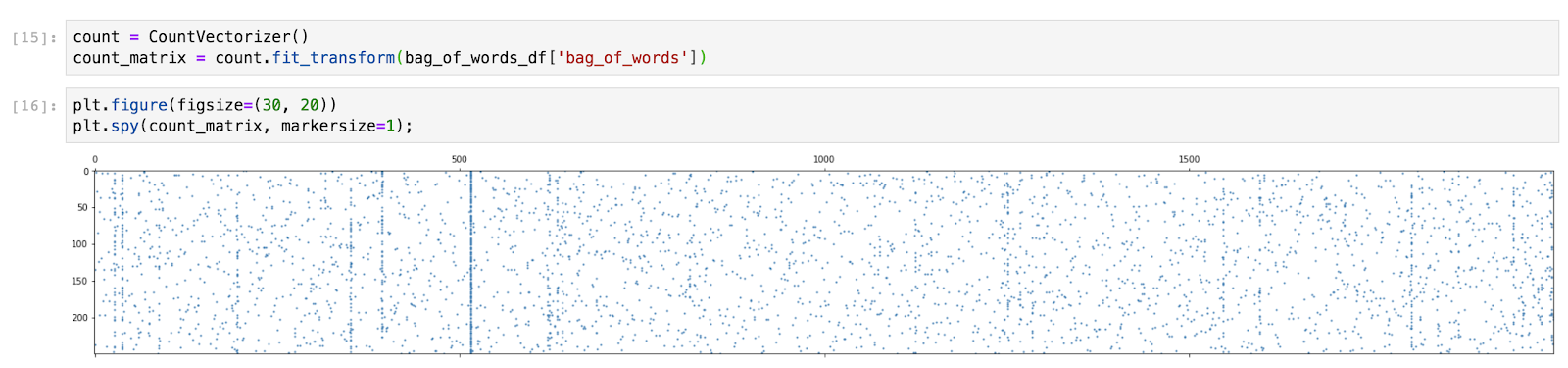```count = CountVectorizer()
count_matrix = count.fit_transform(bag_of_words_df['bag_of_words'])```
```plt.figure(figsize=(30, 20))
plt.spy(count_matrix, markersize=1);```

By feeding count_matrix to the cosine_similarity function, we will have as a result a 250x250 matrix, same number of rows and columns as the number of movies in our data. This matrix contains the values of similarity of each movie to all the other ones in the list. For this reason the matrix has all 1’s on the diagonal (each movie is perfectly similar to itself), and it’s symmetrical (movie A is similar to movie B the same way movie B is similar to movie A).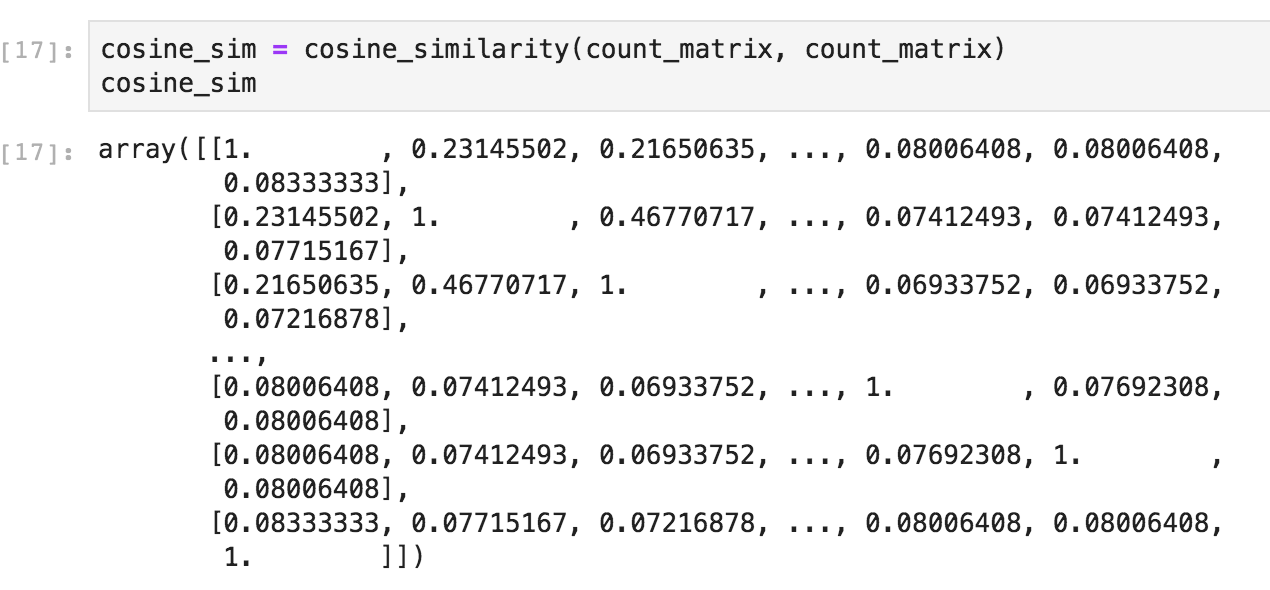```cosine_sim = cosine_similarity(count_matrix, count_matrix)
cosine_sim```

Now that we have our similarity values, we are ready to write a function that will take a title as input and return the n most similar movies.

## 4. Creating and testing the recommender function

This function detects the movie numerical index from the title, and uses the same index to access the cosine similarity matrix at the corresponding row. Then, it sorts the similarity values for that specific row discarding the first one (which will always be 1), and gets the indexes of the most similar n movies to return their titles.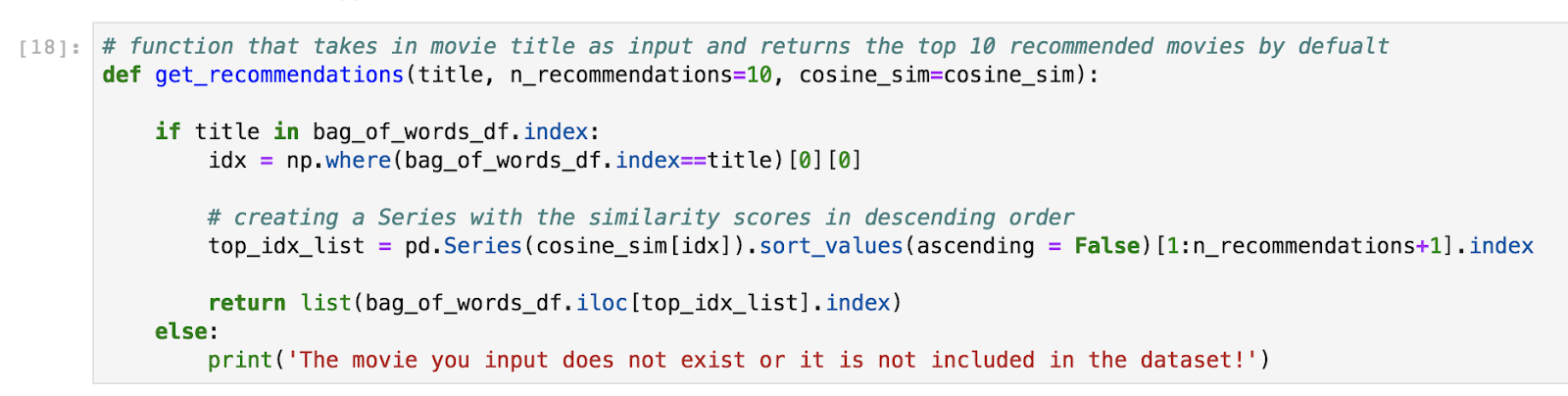```def get_recommendations(title, n_recommendations=10, cosine_sim=cosine_sim):

if title in bag_of_words_df.index:
idx = np.where(bag_of_words_df.index==title)

# creating a Series with the similarity scores in descending order
top_idx_list = pd.Series(cosine_sim[idx]).sort_values(ascending = False)[1:n_recommendations+1].index

return list(bag_of_words_df.iloc[top_idx_list].index)
else:
print('The movie you input does not exist or it is not included in the dataset!')```

Let’s try it out to get the top 6 Godfather’s most similar titles!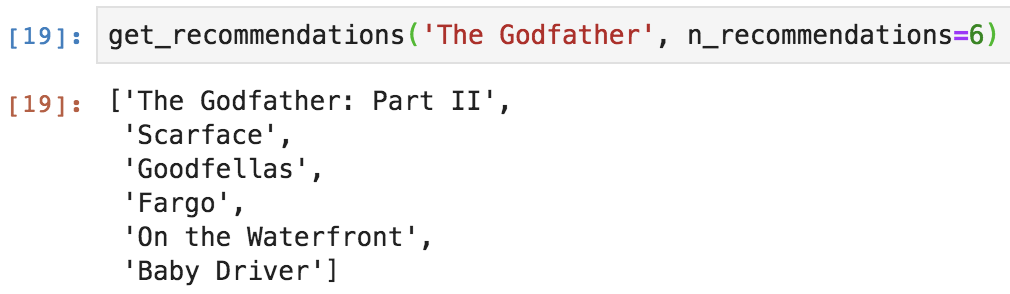`get_recommendations('The Godfather', n_recommendations=6)`

The job is done! Of course this is a pretty limited recommender system because we only used a dataset with 250 movies, but it’s not bad at all! Following the same procedure but using more data, and implementing the key words’ scores for example, this system can be easily refined.

## Ready to Get Started?

Create an account in minutes or connect with our team to learn how Spell can accelerate your business.